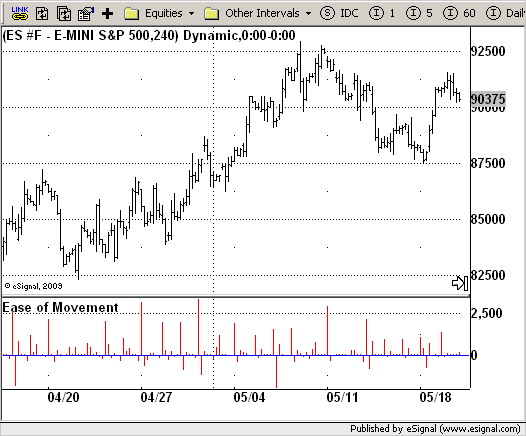# Ease of Movement (EOM)

ICE Data Services -

File Name: EOM.efs

Description:
Ease of Movement (EOM)

Formula Parameters:

Notes:

This indicator gauges the magnitude of price and volume movement.
The indicator returns both positive and negative values where a positive value means the market has moved up from yesterday's value and a negative value means the market has moved down. A large positive or large negative value indicates a large move in price and/or lighter volume. A small positive or small negative value indicates a small move in price and/or heavier volume.
A positive or negative numeric value. A positive value means the market has moved up from yesterday's value, whereas, a negative value means the market has moved down.

EOM.efsEFS Code:

```/*********************************
Provided By:
eSignal (Copyright c eSignal), a division of Interactive Data
Formula Script (EFS) is for educational purposes only and may be
modified and saved under a new file name.  eSignal is not responsible
for the functionality once modified.  eSignal reserves the right
to modify and overwrite this EFS file with each new release.

Description:
Ease of Movement (EOM)

Version:            1.0  05/27/2009

Formula Parameters:                     Default:

Notes:
This indicator gauges the magnitude of price and volume movement.
The indicator returns both positive and negative values where a
positive value means the market has moved up from yesterday's value
and a negative value means the market has moved down. A large positive
or large negative value indicates a large move in price and/or lighter
volume. A small positive or small negative value indicates a small move
in price and/or heavier volume.
A positive or negative numeric value. A positive value means the market
has moved up from yesterday's value, whereas, a negative value means the
market has moved down.

**********************************/
var bInit = false;

function preMain() {
setStudyTitle("Ease of Movement");
setCursorLabelName("EOM", 0);
setDefaultBarFgColor(Color.red,0);
setPlotType(PLOTTYPE_HISTOGRAM);
}

var xEOM = null;

function main() {
var nBarState = getBarState();
var nEOM = 0;
if (bInit == false) {
xEOM = efsInternal("Calc_EOM");
bInit = true;
}
nEOM = xEOM.getValue(0);
if (nEOM == null) return;
return nEOM;
}

var bSecondInit = false;
var xHigh = null;
var xLow = null;
var xVolume = null;
var xHalfRange = null;
var xMidpointMove = null;

function Calc_EOM() {
var nRes = 0;
var nHigh = 0;
var nLow = 0;
var nVolume = 0;
var nBoxRatio = 0;
var nHalfRange = 0;
var nMidpointMove = 0;
var nMidpointMove_1 = 0;
if (bSecondInit == false) {
xHigh = high();
xLow = low();
xVolume = volume();
xHalfRange = efsInternal("Calc_HalfRange");
xMidpointMove = mom(1, xHalfRange);
bSecondInit = true;
}
nHigh = xHigh.getValue(0);
nLow = xLow.getValue(0);
nVolume = xVolume.getValue(0);
nHalfRange = xHalfRange.getValue(0);
nMidpointMove = xMidpointMove.getValue(0);
nMidpointMove_1 =  xMidpointMove.getValue(-1);
if (nMidpointMove_1 == null) return;
if ((nHigh - nLow) != 0) {
nBoxRatio = nVolume / (nHigh - nLow);
} else {
nBoxRatio = 0;
}
if (nBoxRatio != 0) {
nRes = 1000000 * ((nMidpointMove - nMidpointMove_1) / nBoxRatio);
} else {
nRes = 0;
}
return nRes;
}

function Calc_HalfRange() {
var nRes = 0;
nRes = (high(0) - low(0)) * 0.5;
return nRes;
} ```